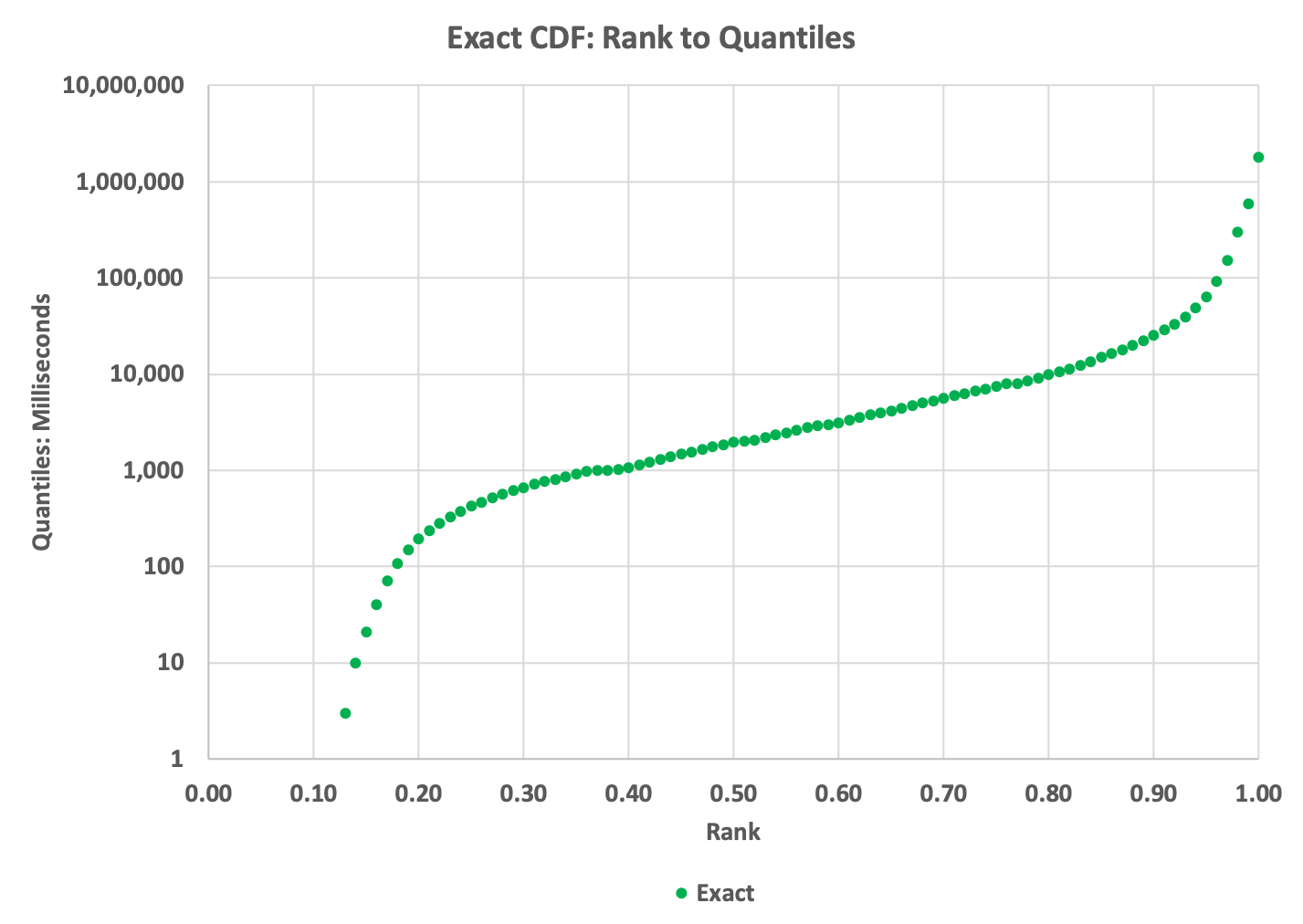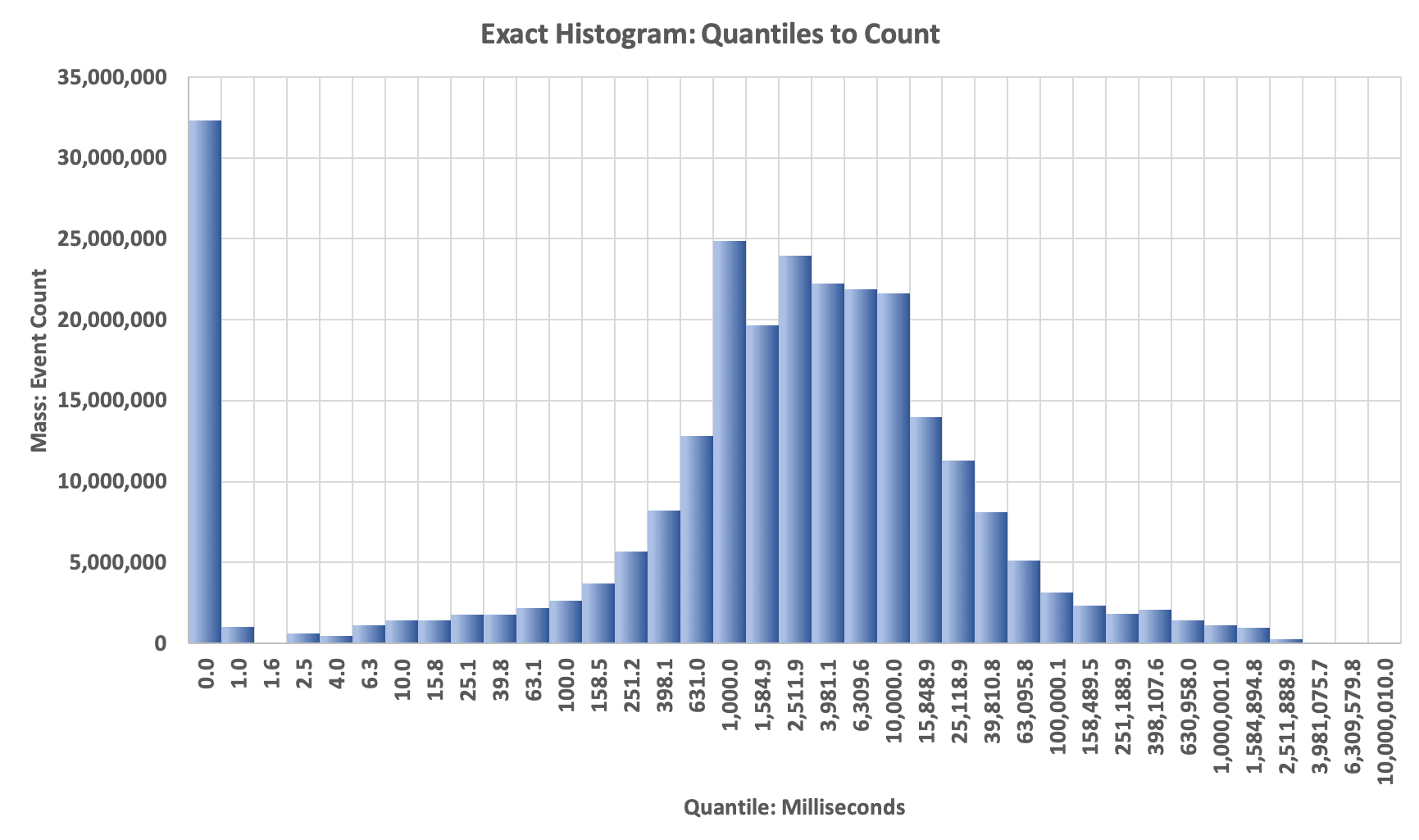# Creating and Plotting The Exact CDF, PMF and Histogram Distributions

In order to measure accuracy of potential algorithms, we need to compute the exact Cumulative Distribution Function (CDF) and Probability Mass Function (PMF) values from a brute-force analysis of the input stream. This, of course, assumes that this is even possible. For very large streams this may not be. Fortunately, our test streams are not so large.

## Prior Knowledge About The Data

Prior to this analysis or even to feed the data into a sketch we do need some basic information about how the data is stored so that we can correctly interpret it.

### StreamA

For our streamA test file we know that the data is stored as consecutive strings of numeric integers separated by a line-feeds.

## 1st Scan

We scan the file to determine the number of items, n in the file. Once this is known we create an internal array of n integers (or longs) that we will use for subsequent processing.

## 2nd Scan

We scan the file again, this time extracting each string value, convert it to a primitive integer and store it into our integer array.

## Sort The Integer Array

The array is sorted and depending on the size of the array, this could take some time.

## 3rd Scan

Now that the array is sorted, the index of every item in the array is also its natural rank. The normalized rank is just the natural rank divided by n. We choose, somewhat arbitrarily, to select the percentile ranks in order to understand what kind of distribution we are dealing with. We scan the array and save the 101 quantile values corresponding to this linear series of ranks: (0, .01, .02, … .99, 1.00).

## Plot The StreamA CDF

This allows us to plot the rank to quantile cumulative distribution or CDF. This is normally plotted with ranks (0 to 1.0) on the X-axis and the 101 quantile values on the Y-axis. This is a very important plot as it tells us a great deal about our data:

• The min and max values
• The dynamic range of the data:
• Does the data have zeros or negative values as well as positive values?
• Do the values span multiple orders-of-magnitude.
• Are there any apparent gaps or jumps in the values?

### The Exact CDFThe reason that the left part of the curve stops at about (0.13, 3) is because slightly less than 13% of the values in the data are zeros, and we can’t plot zeros on the logarithmic Y axis; thus the apparent gap.

## Examine The CDF To Determine Split-Points

This is an important step and determines the quality and value of our PMF or Histogram plot. By examining the above CDF we can choose the aspects of the data that we are interested in by selecting appropriate split-point values, which are the boundaries of the bins of the PMF. This requires some judgement and could be automated, but we did not bother to do this.

For our streamA, we decided that an exponential power series with 5 equally-spaced points per order-of-magnitude should give us a reasonable-looking PMF plot. Based on the min and max values from the CDF, we generated this series mathematically.

## 4th Scan

We scan the array and save the natural ranks (indices) at the chosen split-point values. The difference between adjacent natural ranks is the mass (the number of items) between the respective splitpoints.

## Plot The StreamA Histogram

We can plot the Histogram with the split-point values on the X-axis and the differences between the respective natural ranks on the Y-axis. If we plotted the differences between the respective normalized ranks on the Y-axis we would have the PMF.

### The Exact HistogramOn this plot you will observe the big spike on the left. This represents the mass of all the zero values in the data. This is a bar-chart plot, so the X-axis labels are whatever we assign to them. Because all the bars are the same width, it gives the apperance that the x-Axis is a logarithmic axis … but the plotting software does not know that. Normally, one cannot plot a zero on a logarithmic axis, but here we can get away with it. :)

## Source

You can study the code that implements the above process and generated the above plots.

Run the above profilers as a java application and supply the config file as the single argument. The program will check if the data file has been unzipped, and if not it will unzip it for you.